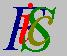Mauro Murzi's pages on Philosophy of Science - Quantum mechanics
A brief recapitulationSecond Schrödinger equation Tunnel effect# [3. Schrödinger equations.]

## A brief recapitulation.

A quantum state is described by a complex function Ψ. Every physical quantity is defined by a quantum operator which satisfies the first Schrödinger equation. The eigenvalues determine the possible value of the quantity. The function Ψ is represented as a sum of the eigenfunctions; the expansion coefficients give the probability of every possible value of the quantity. The time dependence of Ψ is described by the second Schrödinger equation, which determines Ψ at every time. Thus the probability of every admissible value of every physical quantity is determined.Second Schrödinger equation Tunnel effect